##### Chemistry Workbook For Dummies with Online PracticeFor the chemist, Hess's law is a valuable tool for dissecting heat flow in complicated, multistep reactions. For the confused or disgruntled chemistry student, Hess's law is a breath of fresh air. In essence, the law confirms that heat behaves the way we'd like it to behave: predictably.

Imagine that the product of one reaction serves as the reactant for another reaction. Now imagine that the product of the second reaction serves as the reactant for a third reaction. What you have is a set of coupled reactions, connected in series like the cars of a train: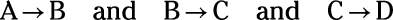Therefore,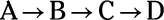You can think of these three reactions adding up to one big reaction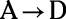What is the overall enthalpy change associated with this reaction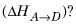Here's the good news: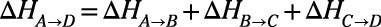Enthalpy changes are additive. But the good news gets even better. Imagine that you're trying to figure out the total enthalpy change for the following multistep reaction: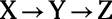Here's a wrinkle: For technical reasons, you can't measure this enthalpy change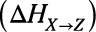directly but must calculate it from tabulated values for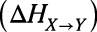and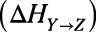No problem, right? You simply look up the tabulated values and add them. But here's another wrinkle: when you look up the tabulated values, you find the following: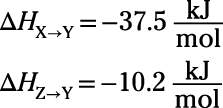Gasp! You need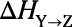but you're provided only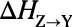Relax. The enthalpy change for a reaction has the same magnitude and opposite sign as the reverse reaction. So if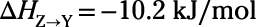then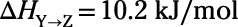It really is that simple: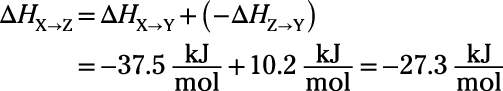Thanks be to Hess.

Try an example. Calculate the reaction enthalpy for the following reaction: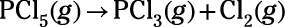Use the following data: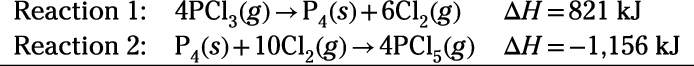Reaction enthalpies are given for two reactions. Your task is to manipulate and add Reactions 1 and 2 so the sum is equivalent to the target reaction. First, reverse Reactions 1 and 2 to obtain Reactions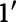and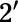and add the two reactions. Identical species that appear on opposite sides of the equations cancel out (as occurs with species P4 and Cl2):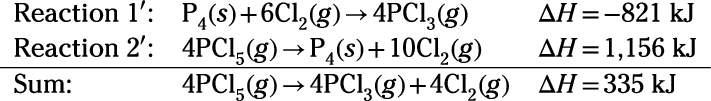Finally, divide the sum by 4 to yield the target reaction equation: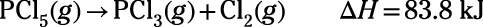So, the reaction enthalpy for the reaction is 83.8 kJ.